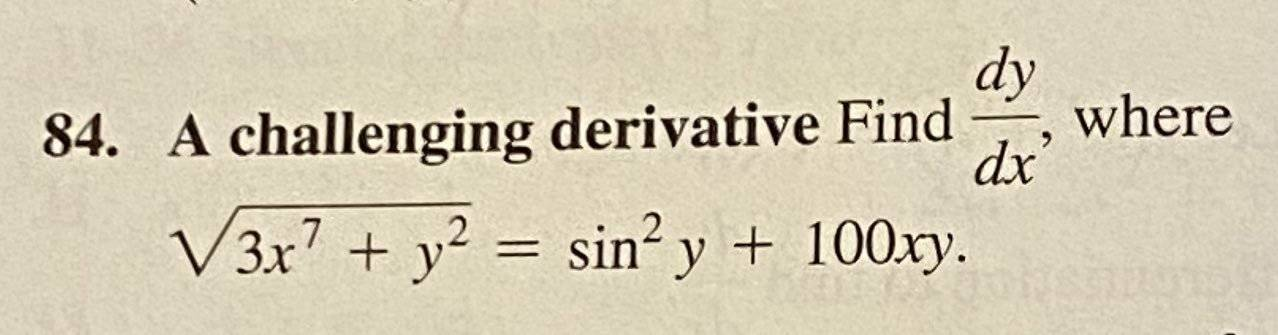# Don't solving this problem, just need some info.

Nate LearningShould I start off by squaring both sides to get rid of the radical on the left? and then start the derivative process? Thank you.

skeeter
Looking at the square of the right side, i probably would not.

Gold Member
MHB
View attachment 11217

Should I start off by squaring both sides to get rid of the radical on the left? and then start the derivative process? Thank you.
You certainly could do it that way, but I don't see that it would be any easier than just differentiating the equation as it stands. Write it as $$\bigl(3x^7 + y^2\bigr)^{1/2} = \sin^2y + 100xy,$$ and differentiate both sides with respect to $x$ using the chain rule.

jonah1
Beer induced query follows.
View attachment 11217

Should I start off by squaring both sides to get rid of the radical on the left? and then start the derivative process? Thank you.
From which book did you get this challenging derivative?

HOI
$(3x^7+ y^2)^{1/2}= sin^2(y)+ 100xy$

The derivative of $3x^7+ y^2$ with respect to x is $21x^6+ 2y\frac{dy}{dx}$ so the derivative of $(3x^7+ y^2)^{1/2}$ is $\frac{1}{2}(3x^7+ y^2)^{-1/2}(21x^6+ 2y\frac{dy}{dx})$.

The derivative of $sin^2(y)$ with respect to x is $2 sin(y) cos(y)\frac{dy}{dx}$.

And the derivative of $100 xy$ with respect to x is $100y+ 100x\frac{dy}{dx}$.

So the derivative of $(3x^7+y^2)^{1/2}= sin^2(y)+ 100xy$ with respect to x is $\frac{1}{2}(3x^7+ y^2)^{-1/2}(21x^6+ 2y\frac{dy}{dx})= 2 sin(y) cos(y)\frac{dy}{dx}+ 100y+ 100x\frac{dy}{dx}$.

Solve that equation for $\frac{dy}{dx}$.